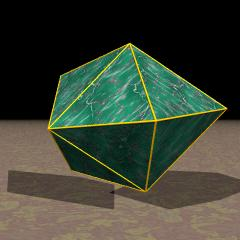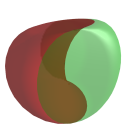Acronym snadow, J84 Name snub disphenoid,snub digonal antiprism `© ©` Vertex figure ,  Coordinates (0, ±1/2, p) (±r, 0, q) (0, ±r, -q) (±1/2, 0, -p) ```where r = 0.644584 is a root of r3-3r2/2-r+1=0 p = [sqrt(3/4+r-r2)+sqrt(3/4-r2)]/2 = 0.783931, q = [sqrt(3/4+r-r2)-sqrt(3/4-r2)]/2 = 0.205562 ``` General of army (is itself convex) Colonel of regiment (is itself locally convex) Confer uniform relative: ike   related Johnson solids: snisquap   general polytopal classes: deltahedra   Johnson solids ExternallinksThe provided coordinates show that snadow could be encased into a square brick A r r, where r is as given above and A=2p. Moreover the equatorial zick-zack of edges and the polar digons would be completely incident to the faces of that brick.

Incidence matrix

```xoBo oBox&#xt   → outer heights = p-q = 0.578369
inner height = 2q = 0.411123
where B = 2r = 1.289169 (pseudo)

o... o...    & | 4 * | 1 2 1 0 | 2 2  
.o.. .o..    & | * 4 | 0 2 1 2 | 1 4  
---------------+-----+---------+----
x... ....    & | 2 0 | 2 * * * | 2 0  digons
oo.. oo..&#x & | 1 1 | * 8 * * | 1 1
o.o. o.o.&#x & | 1 1 | * * 4 * | 0 2
.oo. .oo.&#x   | 0 2 | * * * 4 | 0 2  equatorials
---------------+-----+---------+----
xo.. ....&#x & | 2 1 | 1 2 0 0 | 4 *
ooo. ooo.&#x & | 1 2 | 0 1 1 1 | * 8
```